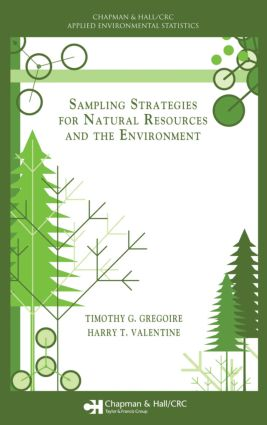Sampling Strategies for Natural Resources and the Environment

1st Edition

Chapman and Hall/CRC

494 pages | 85 B/W Illus.

Purchasing Options:\$ = USD
Hardback: 9781584883708
pub: 2007-07-12
SAVE ~\$24.00
\$120.00
\$96.00
x
eBook (VitalSource) : 9780429095528
pub: 2007-07-12
from \$28.98

FREE Standard Shipping!

Description

Written by renowned experts in the field, Sampling Strategies for Natural Resources and the Environment covers the sampling techniques used in ecology, forestry, environmental science, and natural resources. The book presents methods to estimate aggregate characteristics on a per unit area basis as well as on an elemental basis. In addition to common sampling designs such as simple random sampling and list sampling, the authors explore more specialized designs for sampling vegetation, including randomized branch sampling and 3P sampling.

One of the book's unique features is the emphasis on areal sampling designs, including plot/quadrat sampling, Bitterlich sampling, line intersect sampling, and several lesser known designs. The book also provides comprehensive solutions to the problem of edge effect. Another distinguishing aspect is the inclusion of sampling designs for continuums, focusing on the methods of Monte Carlo integration.

By presenting a conceptual understanding of each sampling design and estimation procedure as well as mathematical derivations and proofs in the chapter appendices, this text promotes a deep understanding of the underpinnings of sampling theory, estimation, and inference. Moreover, it will help you reliably sample natural populations and continuums.

Reviews

This book was written by renowned experts in the field, and thus it is no surprise that they did an excellent job … The bibliography is very comprehensive, and the subject and author indexes are well designed. Numerous examples and exercises illustrate the theoretical developments … I am convinced that this book is absolutely worth having.

—Daniel Mandallaz, JASA, 2008

I expect to use Sampling Strategies for Natural Resources and the Environment for some of my environmental science and statistics classes and as a reliable professional reference for many years.

—Anthony J. Sadar, Pollution Equipment News, 2007

PREFACE

INTRODUCTION

The need for sampling strategies

A medley of sampling scenarios

Probability sample

Inference

Population descriptive parameters

Historical note

Terms to remember

Appendix

SAMPLING DISTRIBUTION OF AN ESTIMATOR

Distribution of values

Estimation

Interval estimation

The role of simulated sampling

Other considerations

Terms to remember

Exercises

Appendix

SAMPLING DESIGNS FOR DISCRETE POPULATIONS

Introduction

Equal probability designs

Unequal probability designs

Terms to remember

Exercises

Appendix

SAMPLING DESIGNS FOR CONTINUOUS POPULATIONS

Introduction

Crude Monte Carlo

Importance sampling

Control variate estimation

Sampling in two or three dimensions

General notation

Terms to remember

Exercises and projects

Appendix

STRATIFIED SAMPLING DESIGNS

Introduction

Rationale for stratified sampling

Estimation with stratified sampling

Sample allocation among strata

Incorrect assignment of population elements into strata

Double sampling for stratification

Poststratification

Stratified sampling of a continuous population

Terms to Remember

Exercises

Appendix

USING AUXILIARY INFORMATION TO IMPROVE ESTIMATION

Generalized ratio estimator

Bias of the generalized ratio estimator

Variance of the generalized ratio estimator

Estimated variance of the generalized ratio estimator

Confidence interval estimation

Ratio estimation with systematic sampling design

Generalized ratio estimation with stratified sampling

Generalized regression estimator

Double sampling with ratio and regression estimation

Terms to Remember

Exercises

Appendix

SAMPLING WITH FIXED AREA PLOTS

Introduction

Notation

Sampling protocol

Estimation

Edge effect

Plot size and shape

Estimating change

Terms to remember

Exercises

Appendix

BITTERLICH SAMPLING

Introduction

Fundamental concepts

Estimation following Bitterlich sampling

Edge effect

Double sampling

Sampling to estimate change in stock

Terms to remember

Exercises

LINE INTERSECT SAMPLING

Introduction

LIS with straight-line transects

Unit area estimators

Estimation with an auxiliary variate

Estimating the mean attribute

Nesting transects of different lengths

Dealing with edge effect in LIS

Transects with multiple segments

Parallel transects of uneven length

Terms to remember

Exercises

Appendix

A MONTE CARLO INTEGRATION APPROACH TO AREAL SAMPLING

Areal sampling

Plot sampling

Bitterlich sampling

Point relascope sampling

Line intersect sampling

Perpendicular distance sampling

Edge correction

Redux: Continuous versus discrete

Terms to Remember

Exercises

Appendix

MISCELLANEOUS METHODS

Introduction

Point relascope sampling

Horizontal line sampling

Transect relascope sampling

Ranked set sampling

3P sampling

Terms to Remember

Exercises

Appendix

TWO-STAGE SAMPLING

Customary two-stage sampling

General two-stage sampling

Three-stage sampling

Terms to Remember

RANDOMIZED BRANCH SAMPLING

Terminology

Path selection

Estimation

Selection probabilities

Tools and tricks of the trade

Subsampling a path

Terms to remember

Exercises and projects

Appendix

SAMPLING WITH PARTIAL REPLACEMENT

Introduction

Estimation with partially replaced sampling units

Estimation of change

SPR with stratification

SPR for three occasions

Concluding remarks

Terms to remember

Appendix

BIBLIOGRAPHY

INDEX